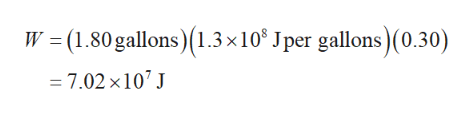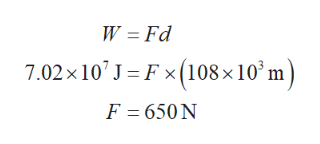Suppose a car travels 108 km at a speed of 35.0 m/s, and uses 1.80 gallons of gasoline. Only 30% of the gasoline goes into useful work by the force that keeps the car moving at constant speed despite friction. (The energy content of gasoline is 1.3 ✕ 108 J per gallon.)(a) What is the force exerted to keep the car moving at constant speed?  N(b) If the required force is directly proportional to speed, how many gallons will be used to drive 108 km at a speed of 28.0 m/s?  gallons

Question

Suppose a car travels 108 km at a speed of 35.0 m/s, and uses 1.80 gallons of gasoline. Only 30% of the gasoline goes into useful work by the force that keeps the car moving at constant speed despite friction. (The energy content of gasoline is 1.3 ✕ 108 J per gallon.)(a) What is the force exerted to keep the car moving at constant speed?
N

(b) If the required force is directly proportional to speed, how many gallons will be used to drive 108 km at a speed of 28.0 m/s?
gallons

Step 1

(a). The amount of work done to keep the car moving at constant speed can be calculated as follows,help_outlineImage TranscriptioncloseW (1.80 gallons)1.3 x 10 Jper gallons)(0.30) - 7.02 x107 J fullscreen
Step 2

Write the expression for work done, substitute the corresponding values, an...help_outlineImage TranscriptioncloseW Fd 7.02x 10 J Fx (108x10 m) F 650N fullscreen

Want to see the full answer?

See Solution

Want to see this answer and more?

Our solutions are written by experts, many with advanced degrees, and available 24/7

See Solution
Tagged in

Physics